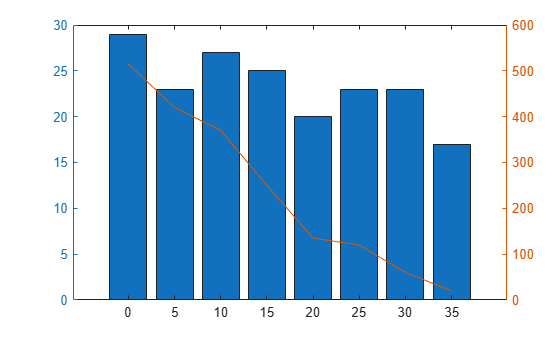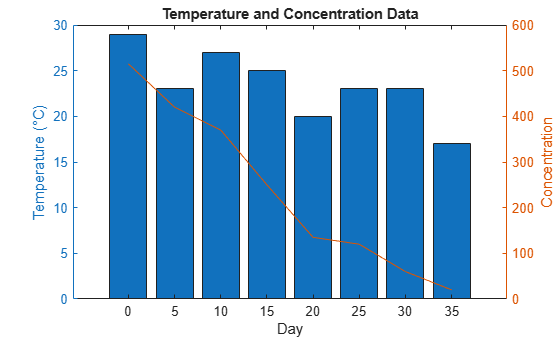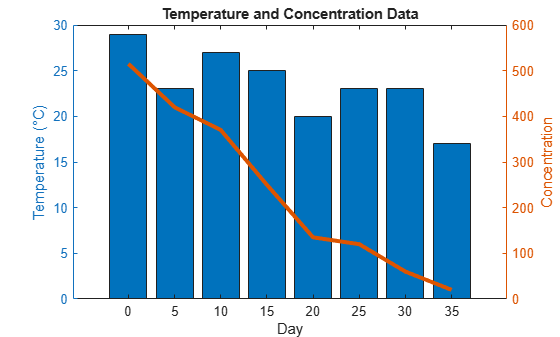# Combine Line and Bar Charts Using Two y-Axes

This example shows how to combine a line chart and a bar chart using two different y-axes. It also shows how to customize the line and bars.

Create a chart that has two y-axes using `yyaxis`. Graphics functions target the active side of the chart. Control the active side using `yyaxis`. Plot a bar chart using the left y-axis. Plot a line chart using the right y-axis. Assign the bar series object and the chart line object to variables.

```days = 0:5:35; conc = [515 420 370 250 135 120 60 20]; temp = [29 23 27 25 20 23 23 17]; yyaxis left b = bar(days,temp); yyaxis right p = plot(days,conc);```Add a title and axis labels to the chart.

```title('Temperature and Concentration Data') xlabel('Day') yyaxis left ylabel('Temperature (\circC)') yyaxis right ylabel('Concentration')```Change the width of the chart line and change the bar colors.

```p.LineWidth = 3; b.FaceColor = [ 0 0.447 0.741];```